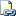# DATEDIFF (Transact-SQL)

Returns the number of date and time boundaries crossed between two specified dates.Transact-SQL Syntax Conventions

## Syntax

``````DATEDIFF ( datepart , startdate , enddate )
``````

## Arguments

• datepart
Is the parameter that specifies on which part of the date to calculate the difference. The following table lists dateparts and abbreviations recognized by SQL Server 2005. These dateparts and abbreviations cannot be supplied as a user-declared variable.

Datepart Abbreviations

year

yy, yyyy

quarter

qq, q

month

mm, m

dayofyear

dy, y

day

dd, d

week

wk, ww

Hour

hh

minute

mi, n

second

ss, s

millisecond

ms

• startdate -
Is the starting date for the calculation. startdate is an expression that returns a datetime or smalldatetime value, or a character string in a date format.

Because smalldatetime is accurate only to the minute, when a smalldatetime value is used, seconds and milliseconds are always 0.

If you specify only the last two digits of the year, values less than or equal to the last two digits of the value of the two-digit year cutoff configuration option are in the same century as the cutoff year. Values greater than the last two digits of the value of this option are in the century that comes before the cutoff year. For example, if the two-digit year cutoff is 2049 (default), 49 is interpreted as 2049 and 2050 is interpreted as 1950. To avoid ambiguity, use four-digit years.

• enddate
Is the ending date for the calculation. enddate is an expression that returns a datetime or smalldatetime value, or a character string in a date format.

integer

## Remarks

startdate is subtracted from enddate. If startdate is later than enddate, a negative value is returned.

DATEDIFF produces an error if the result is out of range for integer values. For milliseconds, the maximum number is 24 days, 20 hours, 31 minutes and 23.647 seconds. For seconds, the maximum number is 68 years.

The method of counting crossed boundaries such as minutes, seconds, and milliseconds makes the result specified by DATEDIFF consistent across all data types. The result is a signed integer value equal to the number of datepart boundaries crossed between the first and second date. For example, the number of weeks between Sunday, January 4, and Sunday, January 11, is 1.

## Examples

The following example determines the difference in days between the current date and the order date for products in the `AdventureWorks` database.

``````USE AdventureWorks;
GO
SELECT DATEDIFF(day, OrderDate, GETDATE()) AS NumberOfDays